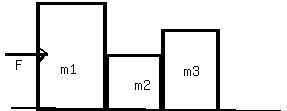# Question# The figure shows 3 crates being pushed over a concrete floor by a horizontal force f of magnitude 440N.

Other
ANSWEREDThe figure shows 3 crates being pushed over a concrete floor by a horizontal force f of magnitude 440N. The masses of the cratesare $$\displaystyle{m}_{{1}}={30}$$ kg, $$\displaystyle{m}_{{2}}={10}$$ kg, and $$\displaystyle{m}_{{3}}={20}$$ kg.The coefficient of kineticfriction between the floor and each of the crates is 0.7. a) what is the magnitude $$\displaystyle{F}_{{{32}}}$$ of the force on crate 3 from crate 2? b) If the crates then slide onto a polished floor, where the coefficientof kinetic friction is less than 0.700, is magnitude $$F_{32}$$ more than,less than, or the same as it was when the coeffient was 0.700?2021-03-26
$$\displaystyle{m}_{{1}}$$
F pushing right
friction pushing left
$$\displaystyle{m}_{{2}}:$$
$$\displaystyle{m}_{{1}}$$ pushing right
$$\displaystyle{m}_{{3}}$$ pushing left
friction pushing left
$$\displaystyle{m}_{{3}}:$$
$$\displaystyle{m}_{{2}}$$ pushing right
friction pushing left
a) $$\displaystyle{F}={m}{a}$$
first we have to find the net acceleration of the system.
$$\displaystyle{F}_{{a}}-{F}_{{f}}={\left({m}_{{1}}+{m}_{{2}}+{m}_{{3}}\right)}{a}$$
$$\displaystyle{F}_{{a}}-\mu{\left({m}_{{1}}+{m}_{{2}}+{m}_{{3}}\right)}{g}={\left({m}_{{1}}+{m}_{{2}}+{m}_{{3}}\right)}{a}$$
$$\displaystyle{a}={\frac{{{F}_{{a}}-\mu{\left({m}_{{1}}+{m}_{{2}}+{m}_{{3}}\right)}}}{{{m}_{{1}}+{m}_{{2}}+{m}_{{3}}}}}$$
After you find the acceleration, multiply it by $$\displaystyle{m}_{{3}}$$ and that wikk be the answer
b) $$\displaystyle{F}_{{{32}}}$$ will be higher before.
In our equation, a smaller $$\displaystyle\mu$$ will result in a large numerator, and a larger acceleration. Since $$\displaystyle{F}={m}{a}$$, a high acceleration will also increase the force.